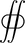## FANDOM

162 Pages

Maxwell's equations are a set of four partial differential equations describing classical electromagnetism. They are:

Maxwell's equations in SI units in vacuum
(for reference)
Name Differential form Integral form
Gauss's law (in vacuum) $\nabla \cdot \vec{\mathbf{E}} = \frac {\rho} {\epsilon_0}$${S }$ $\vec{\mathbf{E}} \cdot \ \mathrm{d} \vec{s} = \frac{q}{\epsilon_0}$
Gauss's law for magnetism $\nabla \cdot \vec{\mathbf{B}} = 0$${S }$ $\vec{\mathbf{B}} \cdot \ \mathrm{d} \vec{s} = 0$
Faraday's law of induction $\nabla \times \vec{\mathbf{E}} = -\frac{\partial \vec{\mathbf{B}}} {\partial t}$ $\oint \vec{\mathbf{E}} \cdot d \vec{r} = - \frac{d}{dt} \iint \vec{\mathbf{B}} \cdot d \vec{s}$
Ampère's circuital law
(with Maxwell's correction)
$\nabla \times \vec{\mathbf{B}} = \mu_0 \ \vec{\mathbf{J}} + \frac{1}{c^2} \ \frac{\partial \vec{\mathbf{E}}} {\partial t}\$ $\oint \vec{\mathbf{B}} \cdot d \vec{r} = \mu_0 \iint \vec{\mathbf{J}} \cdot d \vec{s} + \frac{1}{c^2} \iint \vec{\mathbf{E}} \cdot d \vec{s}$

## Descriptions of each lawEdit

### Gauss's lawEdit

Gauss's law states that the total electric flux passing through any charged surface is proportional to the charge within the surface. In other words, the divergence at any point is equal to charge density over the permittivity.

### Gauss's law for magnetismEdit

The magnetic flux passing through any closed surface is zero, or the divergence is zero everywhere. This means there can be no magnetic monopoles (magnets with only a north or a south pole).

### Faraday's law of inductionEdit

An electric potential can be created by a changing magnetic flux in a closed loop. The negative sign represents Lenz's law.

### Ampère's circuital lawEdit

The line integral of a magnetic field around any closed loop is proportional to the current flowing around the loop and the change in electric flux through the loop (the latter part is called Maxwell's addition). It is related to the speed of light (c) by the formula

$c = \frac{1}{\sqrt{\mu_0 \epsilon_0}}$
Community content is available under CC-BY-SA unless otherwise noted.